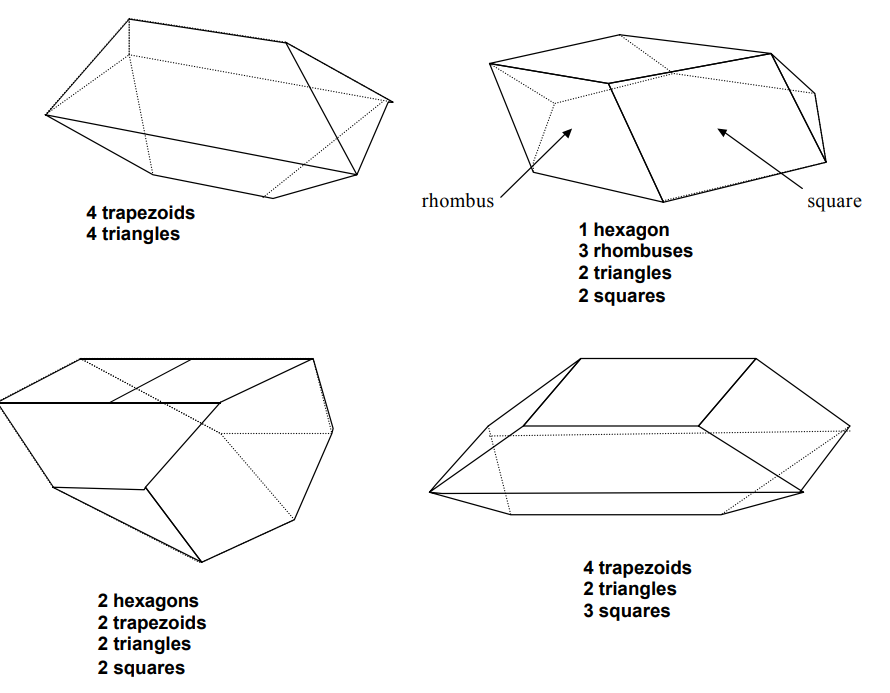Subject:
Mathematics

Topics: Geometry
Common Core State Standard: 6.G.1, 7.G.6,
Concepts:

· Polygon
· Polyhedron

Knowledge and Skills:

· Can identify/describe common polygons.

Materials:

· One model polyhedron for each pair of students.
· Complete set of polygons for each of the model polyhedrons.
· Labeled baskets for different types of polygons.

Lesson:

Procedure: Review the definition of “polygon,” the types of polygons and their characteristics. (Elicit as much of this information from the students as possible.)

Show students models of polyhedrons, and hand out one to each pair of students. Ask them to study them and notice what polygons are used. Make sure that students’
observations include key points (“it’s a solid figure” and “the sides are polygons”) so these points are covered. In your discussion, introduce the word “polyhedron,” discuss
its derivation (“many faces”) and evolve a definition (“a solid figure whose sides are polygons”).

Students are to work in pairs. Tell the students that each pair is to make a copy of their model, using the polygons in the baskets at the front of the room. Once all students
understand the instructions, have them begin.

As students are working, circulate to see how students are doing. As students finish, have them write down 1) how they solved this problem and 2) some possible uses of the